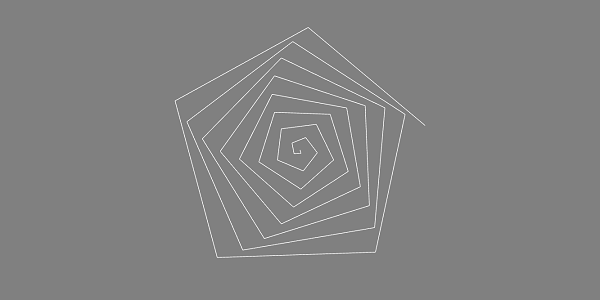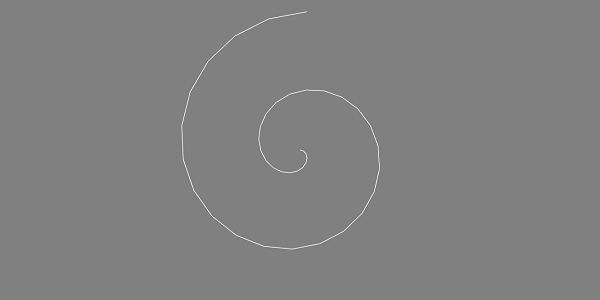# BabylonJS - Line

Line is a basic element in 3D games. To draw a line, you need two points between which you can draw a line.

## Demo

```<!doctype html>
<html>
<meta charset = "utf-8">
<title>BabylonJs - Basic Element-Creating Scene</title>
<script src = "babylon.js"></script>
<style>
canvas {width: 100%; height: 100%;}
</style>

<body>
<canvas id = "renderCanvas"></canvas>
<script type = "text/javascript">
var canvas = document.getElementById("renderCanvas");
var engine = new BABYLON.Engine(canvas, true);
var createScene  = function() {
var scene = new BABYLON.Scene(engine);
scene.clearColor = new BABYLON.Color3( .5, .5, .5);

var camera = new BABYLON.ArcRotateCamera("camera1",  0, 0, 0, new BABYLON.Vector3(0, 0, -0), scene);
camera.setPosition(new BABYLON.Vector3(0, 0, -100));
camera.attachControl(canvas, true);

var light = new BABYLON.HemisphericLight("light1", new BABYLON.Vector3(1, 0.5, 0), scene);
light.intensity = 0.7;

var pl = new BABYLON.PointLight("pl", new BABYLON.Vector3(0, 0, 0), scene);
pl.diffuse = new BABYLON.Color3(1, 1, 1);
pl.specular = new BABYLON.Color3(1, 0, 0);
pl.intensity = 0.95;

// lines creation
var path = [];
for(var t = 0; t < 40; t++) {
var x = t*Math.cos(5*t);
var y = t*Math.sin(5*t);
var z = 0;
path.push(new BABYLON.Vector3(x, y, z));
}

var mesh = BABYLON.Mesh.CreateLines("lines", path, scene, true);

var k = 0;
scene.registerBeforeRender(function() {
pl.position = camera.position;
});
return scene;
};
var scene = createScene();
engine.runRenderLoop(function() {
scene.render();
});
</script>
</body>
</html>
```

## Output

The above line of code generates the following output −To get the coordinates along the x and y axis using the below eqation (maths function) and creating a path with x and y values. The same is given to the createline class of babylonjs. Use any equation of your choice and try creating different lines.

```var mesh = BABYLON.Mesh.CreateLines("lines", path, scene, true);
```

Create a line using the following equation −

```var x = t*Math.cos(5*t);
var y = t*Math.sin(5*t);
var z = 0;
```

Value of t is from 0 to 40.

The shape changes when we execute the following line of code −

```var x = t * Math.cos(6 * t);
var y = t * Math.sin(6 * t);
var z = 0;
```

The shape of line comes as shown below −babylonjs_parametric_shapes.htm